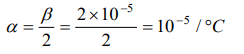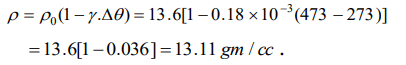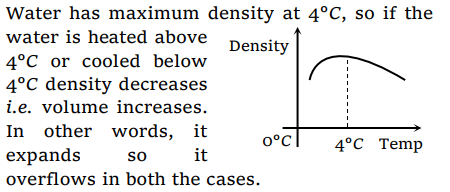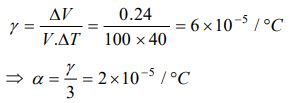## Thermal Properties of Matter Questions and Answers Part-5

1. Water has maximum density at
a) 0°C
b) 32°F
c) – 4°C
d) 4°C

Explanation: Water has maximum density at 4°C

2. At some temperature T, a bronze pin is a little large to fit into a hole drilled in a steel block. The change in temperature required for an exact fit is minimum when
a) Only the block is heated
b) Both block and pin are heated together
c) Both block and pin are cooled together
d) Only the pin is cooled

Explanation: Since coefficient of expansion of steel is greater than that of bronze. Hence with small increase in it's temperature the hole expand sufficiently

3. If the length of a cylinder on heating increases by 2%, the area of its base will increase by
a) 0.5%
b) 2%
c) 1%
d) 4%

Explanation:4. The volume of a gas at 20°C is $100 cm^{3}$  at normal pressure. If it is heated to 100°C, its volume becomes $125 cm^{3}$  at the same pressure, then volume coefficient of the gas at normal pressure is
a) 0.0015/°C
b) 0.0045/°C
c) 0.0025/°C
d) 0.0033/°C

Explanation:0.0033/°C

5. The coefficient of superficial expansion of a solid is $2\times10^{-5}\diagup ^{\circ} C$    . It's coefficient of linear expansion is
a) $4\times10^{-5}\diagup ^{\circ} C$
b) $3\times10^{-5}\diagup ^{\circ} C$
c) $2\times10^{-5}\diagup ^{\circ} C$
d) $1\times10^{-5}\diagup ^{\circ} C$

Explanation:6. Density of substance at 0°C is 10 gm/cc and at 100°C, its density is 9.7 gm/cc. The coefficient of linear expansion of the substance will be
a) $10^{2}$
b) $10^{-2}$
c) $10^{-3}$
d) $10^{-4}$

Explanation:7. Coefficient of real expansion of mercury is $0.18\times10^{-3}\diagup ^{\circ} C$    . If the density of mercury at 0°C is 13.6 gm/cc. its density at 473K is
a) 13.11 gm/cc
b) 26.22 gm/cc
c) 52.11 gm/cc
d) None of these

Explanation:8. The real coefficient of volume expansion of glycerine is 0.000597 per°C and linear coefficient of expansion of glass is 0.000009 per°C. Then the apparent volume coefficient of expansion of glycerine is
a) 0.000558 per°C
b) 0.00057 per°C
c) 0.00027 per°C
d) 0.00066 per°C

Explanation:9. A beaker is completely filled with water at 4°C. It will overflow if
a) Heated above 4°C
b) Cooled below 4°C
c) Both heated and cooled above and below 4°C respectively
d) None of the abovea) $2\times10^{-5}$
b) $6\times10^{-5}$
c) $2.1\times10^{-5}$
d) $1.2\times10^{-5}$